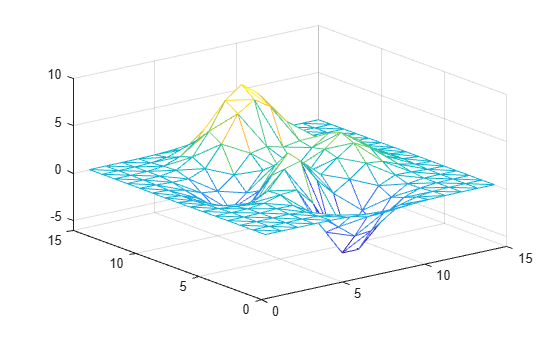# trimesh

Triangular mesh plot

## Syntax

``trimesh(T,x,y)``
``trimesh(T,x,y,z)``
``trimesh(x,y,z,c)``
``trimesh(TO)``
``trimesh(___,Name,Value)``
``h = trimesh(___)``

## Description

````trimesh(T,x,y)` plots the 2-D triangular mesh defined by the points in vectors `x` and `y` and a triangle connectivity matrix `T`.```

example

````trimesh(T,x,y,z)` plots a 3-D triangular mesh.```
````trimesh(x,y,z,c)` also specifies the mesh edge color for a 3-D triangular mesh.```

example

````trimesh(TO)` plots the mesh defined by a 3-D `triangulation` or `delaunayTriangulation` object.```
````trimesh(___,Name,Value)` specifies options using one or more name-value arguments. For example, `trimesh(T,x,y,"LineWidth",2)` sets the edge width to 2 points.```
````h = trimesh(___)` returns a `patch` object used to create the mesh plot. Use `h` to query and modify properties of the plot. For more information, see Patch Properties.```

## Examples

collapse all

Create a set of 3-D points. Compute a Delaunay triangulation connectivity matrix using the `delaunay` function, and plot the mesh.

```[x,y] = meshgrid(1:15,1:15); z = peaks(15); T = delaunay(x,y); trimesh(T,x,y,z)```Alternatively, you can create a `triangulation` object to plot the mesh.

```TO = triangulation(T,x(:),y(:),z(:)); trimesh(TO)```## Input Arguments

collapse all

Triangle connectivity, specified as a 3-column matrix where each row contains the point vertices defining a triangle.

x-coordinates, specified as a column vector.

y-coordinates, specified as a column vector.

z-coordinates, specified as a column vector.

Edge color, specified as a matrix of colormap indices the same size as `z`. For more information, see How Surface Plot Data Relates to a Colormap. For additional control over the surface coloring, use the `"EdgeColor"` and `"FaceColor"` name-value arguments.

Triangulation object, specified as a `triangulation` or `delaunayTriangulation` object.

### Name-Value Arguments

Specify optional pairs of arguments as `Name1=Value1,...,NameN=ValueN`, where `Name` is the argument name and `Value` is the corresponding value. Name-value arguments must appear after other arguments, but the order of the pairs does not matter.

Example: `trimesh(TO,"LineStyle"="--")`

Before R2021a, use commas to separate each name and value, and enclose `Name` in quotes.

Example: `trimesh(TO,"LineStyle","--")`

The properties listed here are only a subset. For a complete list, see Patch Properties.

Face color, specified as a color name, an RGB triplet, or `"none"`.

An RGB triplet is a three-element row vector whose elements specify the intensities of the red, green, and blue components of the color. The intensities must be in the range `[0,1]`; for example, ```[0.4 0.6 0.7]```. This table lists the long and short color name options and the equivalent RGB triplet values.

Long NameShort NameRGB Triplet
`"yellow"``"y"``[1 1 0]`
`"magenta"``"m"``[1 0 1]`
`"cyan"``"c"``[0 1 1]`
`"red"``"r"``[1 0 0]`
`"green"``"g"``[0 1 0]`
`"blue"``"b"``[0 0 1]`
`"white"``"w"``[1 1 1]`
`"black"``"k"``[0 0 0]`

Face transparency, specified as a scalar in the range `[0,1]`. A value of 1 is opaque and 0 is completely transparent. Values between 0 and 1 are semitransparent.

Edge color, specified as a color name, an RBG triplet, or `"none"`. The default color of `[0 0 0]` corresponds to black boundaries.

An RGB triplet is a three-element row vector whose elements specify the intensities of the red, green, and blue components of the color. The intensities must be in the range `[0,1]`; for example, ```[0.4 0.6 0.7]```. This table lists the long and short color name options and the equivalent RGB triplet values.

Long NameShort NameRGB Triplet
`"yellow"``"y"``[1 1 0]`
`"magenta"``"m"``[1 0 1]`
`"cyan"``"c"``[0 1 1]`
`"red"``"r"``[1 0 0]`
`"green"``"g"``[0 1 0]`
`"blue"``"b"``[0 0 1]`
`"white"``"w"``[1 1 1]`
`"black"``"k"``[0 0 0]`

Line width, specified as a positive value in points, where 1 point = 1/72 of an inch. If the line has markers, then the line width also affects the marker edges.

The line width cannot be thinner than the width of a pixel. If you set the line width to a value that is less than the width of a pixel on your system, the line displays as one pixel wide.

Line style, specified as one of the options listed in this table.

Line StyleDescriptionResulting Line
`"-"`Solid line`"--"`Dashed line`":"`Dotted line`"-."`Dash-dotted line`"none"`No lineNo line

## Tips

• To plot a mesh defined by a 2-D `triangulation` or `delaunayTriangulation` object, use the `triplot` function.

## Version History

Introduced before R2006a